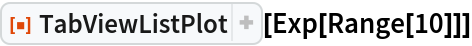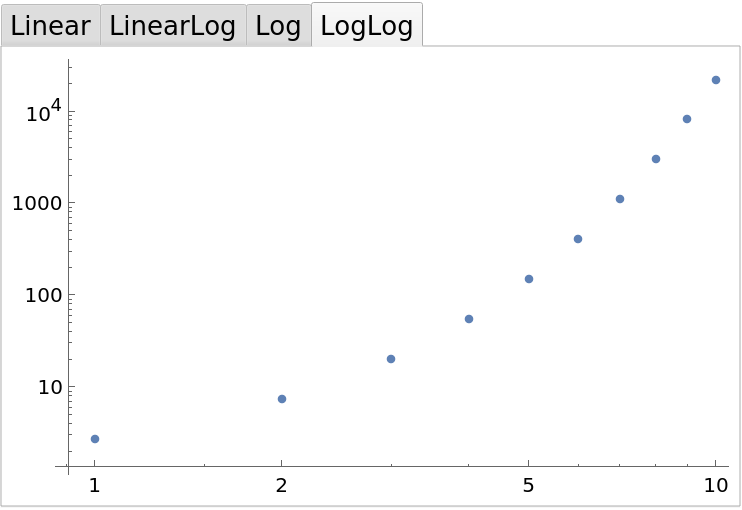Function Repository Resource:

# TabViewListPlot

Create a TabView for a ListPlot using linear and logarithmic axes

Contributed by: Arnoud Buzing
 ResourceFunction["TabViewListPlot"][args] creates a TabView for list plots using linear and logarithmic axes.

## Details and Options

The TabView has four tabs:
 Linear both axes are linear LinearLog x-axis is logarithmic Log y-axis is logarithmic LogLog both axes are logarithmic

## Examples

### Basic Examples (1)

Visualize ex using linear and logarithmic axes:

 In:=Out=Arnoud Buzing

## Version History

• 1.0.0 – 06 April 2020